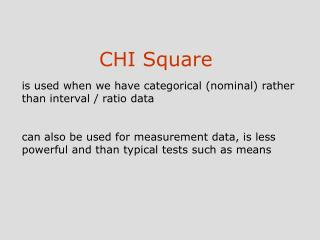DownloadDownload PresentationCHI Square

# CHI Square

Download Presentation## CHI Square

- - - - - - - - - - - - - - - - - - - - - - - - - - - E N D - - - - - - - - - - - - - - - - - - - - - - - - - - -
##### Presentation Transcript

1. is used when we have categorical (nominal) rather than interval / ratio datacan also be used for measurement data, is less powerful and than typical tests such as means CHI Square

2. Measurement data:each number represents a score along a continuumFrequency / Nominal / Categorical Data:Each score represents a frequency in a category.

3. Example: Classify webpage as good or bad Web page seen as Good Bad Total 26 40 68 Question: Is the number of good web pages (26) significantly different than the number of bad webpages (40)?

4. Expected and Observed Frequency Web page seen as Good Bad Total Observed 26 40 68 Expected 34 34 68 Question: Is the number of good web pages (26) significantly different than the number of bad webpages (40)?

5. The CHI square statistic Good Bad Total Observed 26 40 68 Expected 34 34 68 CHI square or X2 = N = 1861.00 X2 = 2.94 Look up the appropriate degrees of freedom in the X2 table E. If X2 bigger than the critical X2, then it is significant

6. CHI Square test has exactly the same logic as a t-testThe only difference is that it is computed on frequencies rather than scores

7. CHI Square for a multicategory case Good Bad Medium Total Observed 26 40 15 81 Expected 27 27 27 81 CHI square or X2 = X2 = 11.63

8. SPSS output NPar Tests Chi-Square Test Frequencies

9. CHI Square for a Contingency Table Analysis (when there is more than one variable) Good Bad Medium Total Finance 26 (27) 40 (27) 15 (27) 81 Newspaper 21 (30) 27 (30) 42 (30) 90 The table shows that webpages in the Finance category were more were more likely to be good than were webpages in the Newspaper condition. Thus, the column a webpage is in (Good, Bad, or Medium) graduate) is contingent upon (depends on) the row the webpage is in (Finance or newspaper category)

10. Contingency Table Analysis Good Bad Medium Total Finance 36 (27) 25 (27) 20 (27) 81 Newspaper 21 (27) 27 (27) 33 (27) 81

11. NPar Tests Chi-Square Test Frequencies SPSS:using the Non Parametric tool

12. SPSS: using cross tabs Crosstabs Mean = 393.2

13. Issues with CHI Square Problem of small expected frequencies Not a good test when expected frequencies are small, i.e., less than 5 Use CHI Square on frequencies not proportions. Convert proportions to frequencies using sample size Minimum = 0 Maximum = 4132

14. How to obtain expected frequenciesUniform DistributionNormal DistributionTheoretical Reason

15. Confidence Limits on Mean • Sample mean is a point estimate • We want interval estimate • Probability that interval computed this way includes m = 0.95

16. For Our Data

17. Confidence Interval • The interval does not include 5.65--the population mean without a violent video • Consistent with result of t test • What can we conclude from confidence interval?